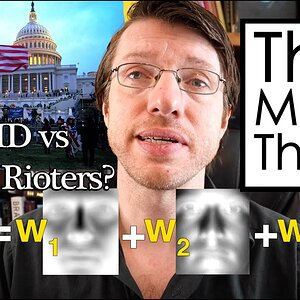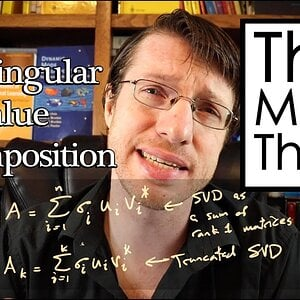# linear algebra Definition and Topics - 420 Discussions

Linear algebra is the branch of mathematics concerning linear equations such as:

a

1

x

1

+

+

a

n

x

n

=
b
,

{\displaystyle a_{1}x_{1}+\cdots +a_{n}x_{n}=b,}
linear maps such as:

(

x

1

,

,

x

n

)

a

1

x

1

+

+

a

n

x

n

,

{\displaystyle (x_{1},\ldots ,x_{n})\mapsto a_{1}x_{1}+\cdots +a_{n}x_{n},}
and their representations in vector spaces and through matrices.Linear algebra is central to almost all areas of mathematics. For instance, linear algebra is fundamental in modern presentations of geometry, including for defining basic objects such as lines, planes and rotations. Also, functional analysis, a branch of mathematical analysis, may be viewed as the application of linear algebra to spaces of functions.
Linear algebra is also used in most sciences and fields of engineering, because it allows modeling many natural phenomena, and computing efficiently with such models. For nonlinear systems, which cannot be modeled with linear algebra, it is often used for dealing with first-order approximations, using the fact that the differential of a multivariate function at a point is the linear map that best approximates the function near that point.

View More On Wikipedia.org

6. ### I General Method for Mapping an Ellipsoid to Unit Sphere

I have been working on a problem for a while and my progress has slowed enough I figured I'd try reaching out for some more experience. I am trying to map a point on an ellipsoid to its corresponding point on a sphere of arbitrary size centered at the origin. I would like to be able to shift any...
7. ### I Generalized eigenvector

Hi, I have a set of ODE's represented in matrix format as shown in the attached file. The matrix A has algebraic multiplicity equal to 3 and geometric multiplicity 2. I am trying to find the generalized eigenvector by algorithm (A-λI)w=v, where w is the generalized eigenvector and v is the...
8. ### I A question on inner products

I have the followinq question: Let ##(,)## be a real-valued inner product on a real vector space ##V##. That is, ##(,)## is a symmetric bilinear map ##(,):V \times V \rightarrow \mathbb{R}## that is non-degenerate Suppose, for all ##v \in V## we have ##(v,v) \geq 0## Now I want to prove that...
9. ### Linear Algebra - LU Factorization

Hello all, I have a problem related to LU Factorization with my work following it. Would anyone be willing to provide feedback on if my work is a correct approach/answer and help if it needs more work? Thanks in advance. Problem: Work:
10.### Lecture 5 - Science, Toys, and the PCA

We open this lecture with a discussion of how advancements in science and technology come from a consumer demand for better toys. We also give an introduction to Principle Component Analysis (PCA). We talk about how to arrange data, shift it, and the find the principle components of our dataset.
11.### Lecture 3 - How SVDs are used in Facial Recognition Software

This video builds on the SVD concepts of the previous videos, where I talk about the algorithm from the paper Eigenfaces for Recognition. These tools are used everywhere from law enforcement (such as tracking down the rioters at the Capitol) to unlocking your cell phone.
12.### Lecture 2 - Understanding Everything from Data - The SVD

In this video I give an introduction to the singular value decomposition, one of the key tools to learning from data. The SVD allows us to assemble data into a matrix, and then to find the key or "principle" components of the data, which will allow us to represent the entire data set with only a few
13. ### Riesz Basis Problem

The reference definition and problem statement are shown below with my work shown following right after. I would like to know if I am approaching this correctly, and if not, could guidance be provided? Not very sure. I'm not proficient at formatting equations, so I'm providing snippets, my...
14. ### Orthogonal Projection Problems?

Summary:: Hello all, I am hoping for guidance on these linear algebra problems. For the first one, I'm having issues starting...does the orthogonality principle apply here? For the second one, is the intent to find v such that v(transpose)u = 0? So, could v = [3, 1, 0](transpose) work?

16. ### Determining value of r that makes the matrix linearly dependent

for problem (a), all real numbers of value r will make the system linearly independent, as the system contains more vectors than entry simply by insepection. As for problem (b), no value of r can make the system linearly dependent by insepection. I tried reducing the matrix into reduced echelon...
17. ### Diagonalizing a matrix given the eigenvalues

The following matrix is given. Since the diagonal matrix can be written as C= PDP^-1, I need to determine P, D, and P^-1. The answer sheet reads that the diagonal matrix D is as follows: I understand that a diagonal matrix contains the eigenvalues in its diagonal orientation and that there must...
18. ### Linear Algebra uniqueness of solution

My guess is that since there are no rows in a form of [0000b], the system is consistent (the system has a solution). As the first column is all 0s, x1 would be a free variable. Because the system with free variable have infinite solution, the solution is not unique. In this way, the matrix is...
19. ### Calculus Good textbook for Mathematics

Hey guys, so I was on this thread on tips for self studding physics as a highschooler with the aim to become a theoretical (quantum) physicist in the future. I myself am a 15 year old who wants to become a theoretical physicist in the future. A lot of people in the thread were saying that...

21. ### Help with linear algebra: vectorspace and subspace

So the reason why I'm struggling with both of the problems is because I find vector spaces and subspaces hard to understand. I have read a lot, but I'm still confussed about these tasks. 1. So for problem 1, I can first tell you what I know about subspaces. I understand that a subspace is a...
22. ### Help with subspaces

Summary:: Properties of subspaces and verifying examples Hi, My textbook gives some examples relating to subspaces but I am having trouble intuiting them. Could someone please help me understand the five points they are attempting to convey here (see screenshot).
23. ### System of equations and solving for an unknown

The first thing I do is making the argumented matrix: Then I try to rearrange to make the row echelon form. But maybe that's what confusses me the most. I have tried different ways of doing it, for example changing the order of the equations. I always end up with ##k+number## expression in...
24. ### Confused with this proof for the Cauchy Schwarz inequality

Im confused as finding the minimum value of lambda is an important part of the proof but it isn't clear to me that the critical point is a minimum
25. ### Change of basis to express a matrix relative to a set of basis matrices

Hello, I am studying change of basis in linear algebra and I have trouble figuring what my result should look like. From what I understand, I need to express the "coordinates" of matrix ##A## with respect to the basis given in ##S##, and I can easily see that ##A = -A_1 + A_2 - A_3 + 3A_4##...
26. ### I Invertible polynomials

0 Let T: P2 (R) → P2 (R) be the linear map defined by T(p(x)) = p''(x) - 5p'(x). Is T invertible ? P2 (R) is the vector space of polynomials of degree 2 or less
27. ### Vector space of linear maps

Solution 1. Based on my analysis, elements of ##V## is a map from the set of numbers ##\{1, 2, ..., n\}## to some say, real number (assuming ##F = \mathbb{R}##), so that an example element of ##F## is ##x(1)##. An example element of the vector space ##F^n## is ##(x_1, x_2, ..., x_n)##. From...
28. ### I Zero-point energy of the harmonic oscillator

First time posting in this part of the website, I apologize in advance if my formatting is off. This isn't quite a homework question so much as me trying to reason through the work in a way that quickly makes sense in my head. I am posting in hopes that someone can tell me if my reasoning is...
29. ### Linear algebra inner products, self adjoint operator,unitary operation

b) c and d): In c) I say that ##L_h## is only self adjoint if the imaginary part of h is 0, is this correct? e) Here I could only come up with eigenvalues when h is some constant say C, then C is an eigenvalue. But I' can't find two. Otherwise does b-d above look correct? Thanks in advance!
30. ### I Proving linear independence of two functions in a vector space

Hello, I am doing a vector space exercise involving functions using the free linear algebra book from Jim Hefferon (available for free at http://joshua.smcvt.edu/linearalgebra/book.pdf) and I have trouble with the author's solution for problem II.1.24 (a) of page 117, which goes like this ...